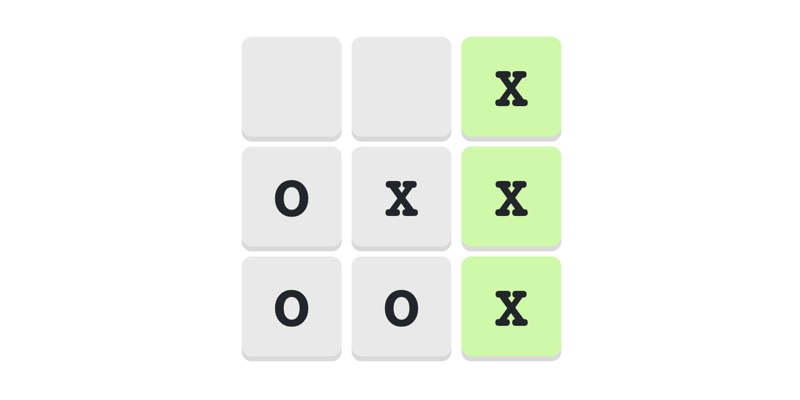# Creating Tic Tac Toe game

In this blog post we will explore the logic used to create a simple Tic Tac Toe game. Feel free to code it in any programming language you like.

GameProgramming

Welcome to my another blog post. In this post we will try to create a simple logic that a computer will use to play Tic Tac Toe game.

## What is Tic Tac Toe game?

It is a simple paper-and-pencil game that involves two players. Total four lines are drawn, two lines are draw perpendicularly and two lines are draw vertically, equidistant from each other which gives us 9 squares in total. Whoever manages to get their mark first in any one of the three rows or any one of the three columns or any one of the two diagonals wins the game.

Note! Being a very simple game, it is highly likely that experienced players would end the game in a draw.

## The board

The board consists of 3x3, i.e., 9 squares as shown below.

``````  0   1   2  ← column index
0   |   |
---+---+---
1   |   |
---+---+---
2   |   |
↑
row index``````

This can be represented using a 2D array with 3 rows and 3 columns. The numbers at the top represents index of the columns and the numbers on the left represents index of the rows.

The 3 rows can be written in terms of their (row,col) set like the following.

``````{(0,0), (0,1), (0,2)}
{(1,0), (1,1), (1,2)}
{(2,0), (2,1), (2,2)}
``````

Similarly, we can represents the 3 columns like the following.

``````{(0,0), (1,0), (2,0)}
{(0,1), (1,1), (2,1)}
{(0,2), (1,2), (2,2)}
``````

And the 2 diagonals can be represented like the following.

``````{(0,0), (1,1), (2,2)}
{(0,2), (1,1), (2,0)}
``````

## Winning SET

It is the set of (row,col) pair that a player can fill with their mark and win the game.

Following are the 8 winning sets consisting of (row,col) pairs.

``````{(0,0), (0,1), (0,2)}
{(1,0), (1,1), (1,2)}
{(2,0), (2,1), (2,2)}
{(0,0), (1,0), (2,0)}
{(0,1), (1,1), (2,1)}
{(0,2), (1,2), (2,2)}
{(0,0), (1,1), (2,2)}
{(0,2), (1,1), (2,0)}
``````

Whoever fills any one of the above WINNING SET first, wins the game.

## Logic for the computer

We will write three directives for the computer to follow.

1. If any 2 out of the 3 pairs of any of the 8 WINNING SETs is filled with COMPUTER's mark - then the COMPUTER must go for the third pair of that WINNING SET and win the match.
2. Else if, USER is going to win in the next move - then block the user by putting COMPUTER's mark.
3. Else, randomly pick an empty cell and fill it with COMPUTER's mark.

## Demo

Check out the game Tic Tac Toe and see the above logic in action.

## Code snippet

``````const makeBotMove = () => {
// pick a cell that will make bot win
for (let i = 0; i < WINNING_CELLS.length; i++) {
const cellsToCheck = WINNING_CELLS[i];
const numberOfCellsFilledWithBotSymbol = cellsToCheck.filter(idx => cells[idx] === BOT_SYMBOL).length;
if (numberOfCellsFilledWithBotSymbol === 2) {
const emptyCellIdx = cellsToCheck.find(idx => cells[idx] === EMPTY_SYMBOL);
if (emptyCellIdx !== undefined && emptyCellIdx >= 0) {
updateCell(emptyCellIdx);
return;
}
}
}

// pick a cell that will stop user
for (let i = 0; i < WINNING_CELLS.length; i++) {
const cellsToCheck = WINNING_CELLS[i];
const numberOfCellsFilledWithUserSymbol = cellsToCheck.filter(idx => cells[idx] === USER_SYMBOL).length;
if (numberOfCellsFilledWithUserSymbol === 2) {
const emptyCellIdx = cellsToCheck.find(idx => cells[idx] === EMPTY_SYMBOL);
if (emptyCellIdx !== undefined && emptyCellIdx >= 0) {
updateCell(emptyCellIdx);
return;
}
}
}

// randomly pick an empty cell
const emptyIndices = cells.reduce((acc, curr, idx) => {
return curr === EMPTY_SYMBOL ? [...acc, idx] : acc;
}, [] as number[]);
const randomIndex = emptyIndices[Math.floor(Math.random() * emptyIndices.length)];
updateCell(randomIndex);
};``````